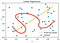# What exactly is an A.I. Model? An ELi5.

Machine Learning Models. We hear about them all the time. They look and sound awesome, but can they predict and react to tough questions? What are they exactly?

Machine Learning can be thought of as “data being parsed through formulas and algorithms”, to give us a result.

The machine learning “model” here would be the relationship between the variables that is calculated based on formulas, algorithms and their relative settings.

For example, a simple Y = mX + c linear function, which shows the relationship between x and y.

Here we have an input X, with an output Y. The “settings” here would be:
m = 1
c = 0.
In very simplistic terms, Output = 1 *Input + 0.

The Model would be to generate this Linear Function from a given data set.

Let’s look at how Machine Learning can generate a linear prediction based on a given data set of plot points.

# Linear Regression

Linear Regression — Linear Regression is the linear modelling of the relationship between variables.

In our school mathematics, this is commonly know as the “line of best fit”.

In this case, the visualization of the model will give us a linear function as seen below.

The data set here is generated by random ( x , y ) coordinates between 0 and 1. There are 40 data points in the above graph.

Using Linear Regression modelling of the random data,
we find that the relationship between the data is a perfect Y = X linear function.

This relationship helps us predict that if
X = 0.5,
Y is most likely 0.5.

## Where is the Machine Learning in this straight line?

When more (non-random) data is put into the data set, the line is likely to shift.

Some changes might be more nuanced than others, especially with big data sets (e.g. 1 million data points).

Machine Learning here is to detect, retrain the model and make predictions and inferences.

You might think “it’s so simple, just redraw the line!”, but what if we have a much more complex task that requires a complex fit?If we want the red squiggly line. Not so straight forward now huh?

Machine Learning, here, will simplify the task of calculating the fit squiggly line!

A more complex task will of course need a more complex model!

# Neural Network Model

An artificial neural network model is based on the calculation of some linear formulas and activation functions with weights, biases (u.e. their “settings”) being adjusted with each calculation.

The above is a 1-layer Neural Network. Each circle is called a neuron, more accurately a perceptron. We can use the above Neural Network model to find the relationships between given data.

We will train a 1-layer NN model with 100 Neurons in the hidden layer.

Let’s see how it fares with the previous data set.

Notice that it gives us the exact same prediction model as the simpler linear regression model.

# How do we choose which model to use?

Simple! Which one is easier to implement, and which one is more accurate? Which one is more suitable for the task at hand?

For a simple problem like the above, using the Linear Regression model is the clear winner!

There are many other Machine Learning models such as Q-learning, Reinforcement Learning, Convolutional Neural Networks and a great many others.

Many are task specific, which mean they are designed to perform better under different “settings” and circumstances.

Written by

Written by

## DJ Wong

#### Certified Ethical Hacker | Consensys Dev Program 2018 | Deeplearning.AI | B.Eng## More From Medium

Welcome to a place where words matter. On Medium, smart voices and original ideas take center stage - with no ads in sight. Watch
Follow all the topics you care about, and we’ll deliver the best stories for you to your homepage and inbox. Explore
Get unlimited access to the best stories on Medium — and support writers while you’re at it. Just \$5/month. Upgrade